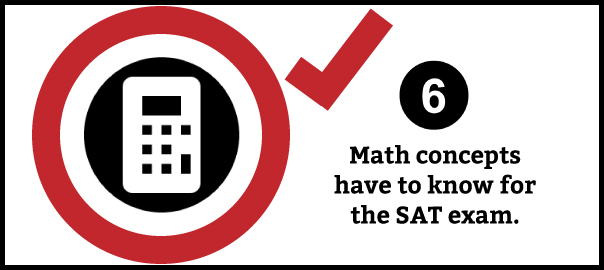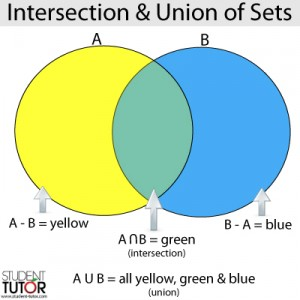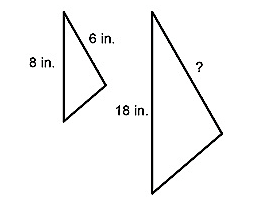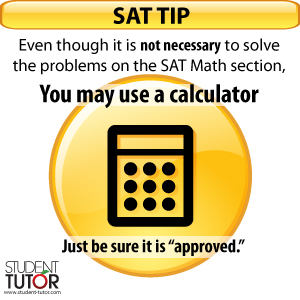# 6 Things You Will See on the SAT Math Section

Master the SAT Math Section by Knowing What is on it!

There are three sections on the SATs: critical reading, writing, and the often dreaded SAT Math section. Dun, dun, dunnnn!

Many parents and students get nervous about the math section especially if a student’s strongest school subject is not mathematics. So let’s dispel some worries by taking a look at what you WILL find on the SAT Math section of the exam.

1. Number and Operations
Here are the sub-categories found in this:

• properties of integers
• arithmetic word problems• number lines
• squares and square roots
• fractions and rational numbers
• elementary number theory (factors, multiples and remainders, prime numbers)
• ratios, proportions, and percentages
• sequences
• sets (union, intersection, elements)
• counting problems
• logical reasoning

2. Algebra and Functions
Here are the sub-categories found in this:

• operations on algebraic expressions
• factoring
• exponents
• evaluating expressions with exponents and roots
• solving equations (determining if an equation has a solution, solving for one variable in terms of another, solving equations involving radical expressions)
• absolute value
• direct translation into mathematical expressions
• inequalities
• systems of linear equations and inequalities
• solving quadratic equations by factoring
• rational equations and inequalities
• direct and inverse variations
• word problems
• functions (notation and evaluation, domain and range, using new definitions, graphs and translations of linear and quadratic functions)

3. Geometry and Measurement
Here are the sub-categories found in this:

• geometric notation
• points and lines
• angles on a plane
• triangles, special right triangles, and the triangle inequality (equilaterals, isosceles, 30-60-90’s, 45-45-90’s, 3-4-5’s, congruent, and similar triangles)• areas, perimeters, and angle measure (squares, rectangles, triangles, parallelograms, other polygons)
• circles (radius, diameter, arcs, tangents, circumference, and area)
• solid geometry (surface area and volume)
• geometric perception
• coordinate geometry (slope, midpoints, using distance formula, parallel and perpendicular lines)
• transformations

4. Data Analysis, Statistics, and Probability
Here are the sub-categories found in this:

• data interpretation
• statistics (mean, median, mode, weighted average, average of algebraic expressions and using averages to find missing values)
• basic probability including geometric probability

5. Questions designed to evaluate how well you use the above concepts to solve real-life math problems
The SAT writers recognize that math and reasoning skills are a huge part of daily life (whether we realize it or not). Additionally, the test’s goal is to find a measure by which college admissions officers can reasonably predict your likelihood of success in college. Therefore, the SAT Math section is packed with problems that will make you evaluate and reason, often in situations that could occur in the “real-world.”6. Problems you can solve WITHOUT a calculator
All problems on the SAT Math section are designed so that it is possible to solve without a calculator.

This is great news! No ‘Law of Cosines’ problems or complicated Matrices! Other good news is that you are allowed to use a calculator to help you move faster through the calculations even though one is not required. Just make sure you bring an “approved” calculator.

Banned calculators are those that talk, can access the internet, or have QWERTY keypads. See official site for more specifics on approved calculators for SAT Math Section.

#### Want to see what WILL NOT appear in SAT Math? Read this quick blog called 8 Things You Won’t See on the SAT Math Section…coming tomorrow!

The following two tabs change content below.#### Laura Petersen

Co-Founder at Student-Tutor
Laura earned a Master's degree in Secondary Education plus Teaching Certification for Mathematics and Psychology in both AZ and CA. Her undergraduate work was in Psychology with a minor in German from UCLA where she graduated magna cum laude. She has been tutoring since the 90's, was a high school teacher 2006-2011, and still teaches for a local college. Laura is passionate about teaching, learning, entrepreneurship, and traveling the world with her husband, their dog Tuck, and her Macbook Pro. -- Join Laura on Linkedin & Twitter!#### Latest posts by Laura Petersen (see all)Subscribe
Notify of